A 400 g block of ice at 0 C is placed inside an empty copper bowl at 30 C”. Given enough time, this system reaches a steady state temperatur

Question

A 400 g block of ice at 0 C is placed inside an empty copper bowl at 30 C”. Given enough time, this system reaches a steady state temperature of 5 C”. If the system is thermally insulated from the surroundings, what is the mass of the copper bowl? The specific heat capacities of water and copper are 4180 J kg K and 386 J kg’K’, respectively. The latent heats of fusion and vaporization of water are 333 kJ kg and 2256 kJ kg’, respectively.

A). 15,6 kg
B). 10.2 kg
C). 17.8 kg
D). 14.7 kg

in progress 0
6 months 2021-07-15T09:28:01+00:00 1 Answers 3 views 0

D.14.7 kg

Explanation:

Mass of block of ice=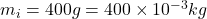1 kg=1000 g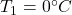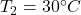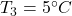Specific heat capacities of water=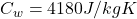Specific heat capacities of copper=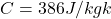Latent heat of fusion=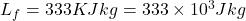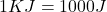Latent heat of vaporization=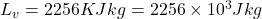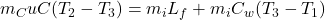Substitute the values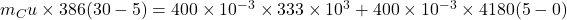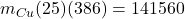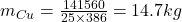Hence, the mass of copper bowl=14.7 kg

Option D is true.